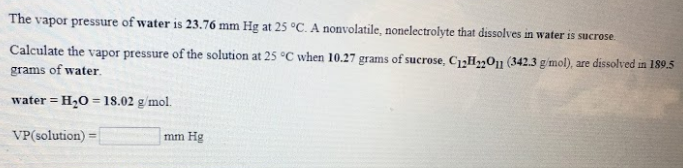# The vapor pressure of water is 23.76 mm Hg at 25 degrees C. A nonvolatile, nonelectrolyte that dissolves in water is sucrose. calculate the vapor pressure of the solution at 25 degrees C when 10.27 grams of sucrose, C12H22O11 (342.3 g/mol) are dissolved in 189.5 grams of water. water = H2O = 18.02 g/mol VP(solution) = ____ mmHg